Like   Tweet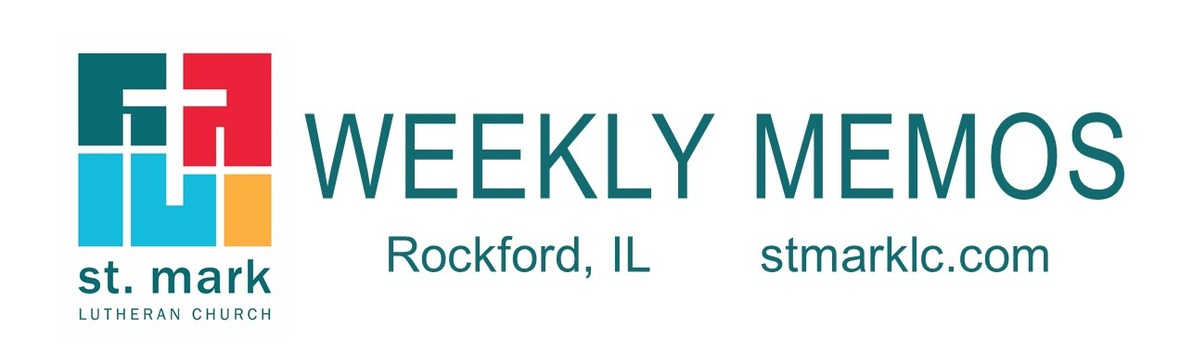table div table+table div table{width:100%;padding:0}table div table+table div table img{width:96.23%;padding:0;float:none}table div table+table div table td{width:100%;padding:0 1.88% 18px}/* styles */## Pastoral ReflectionAn Update on our Pandemic Strategy

Our council, under the guidance of both the ELCA and the Illinois Department of Public Health, created the guidelines we currently have because we want to get back to normal quickly and safely. The guidelines are not meant to restrict us. They exist so that we can move gently away from the pandemic that has held us back for too long. Read more.

 table div table+table+table+table+table div table{width:100%;padding:0}table div table+table+table+table+table div table img{width:96.23%;padding:0;float:none}table div table+table+table+table+table div table td{width:100%;padding:0 1.88% 18px}/* styles */## News and Events

 table div table+table+table+table+table+table+table div table{width:100%;padding:0}table div table+table+table+table+table+table+table div table img{width:96.23%;padding:0;float:none}table div table+table+table+table+table+table+table div table td{width:100%;padding:0 1.88% 18px}/* styles */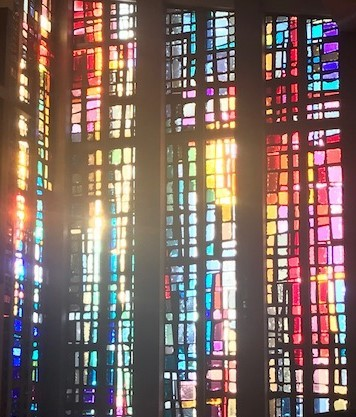You can enjoy Sunday's worship anywhere and anytime after 8:00 A.M on your phone, laptop, iPad, or if you have a smart tv. So grab a cup of coffee or tea, and join our St. Mark family in worship. If you missed a service, they are all there on our YouTube channel.

We will be streaming abbreviated, pre-recorded worship services on our St. Mark YouTube Channel, available for viewing throughout the day on Sundays, beginning at 8:00 A.M.

Look for a special email and push notification from our app on Sunday morning providing a link to this service on YouTube. We hope this is bringing a much-needed sense of community during these difficult times of separation.

 table div table+table+table+table+table+table+table+table+table div table{width:100%;padding:0}table div table+table+table+table+table+table+table+table+table div table img{width:96.23%;padding:0;float:none}table div table+table+table+table+table+table+table+table+table div table td{width:100%;padding:0 1.88% 18px}/* styles */Register for Weekly Wednesday Night Worship here.The service starts at 6:30 p.m.

 table div table+table+table+table+table+table+table+table+table+table+table div table{width:100%;padding:0}table div table+table+table+table+table+table+table+table+table+table+table div table img{width:96.23%;padding:0;float:none}table div table+table+table+table+table+table+table+table+table+table+table div table td{width:100%;padding:0 1.88% 18px}/* styles */Lydia Circle will meet at St. Mark on Thursday, July 30 at 7:00 pm in the cafe. Please wear your mask and bring your bible. We'll be taking temperatures, signing waivers, and social distancing per the St. Mark Covid-19 Strategy and Guidelines. If you can't attend in person or don't feel comfortable attending in person, you can join us by Zoom. Email Andrea for the Zoom Meeting invite.

We will be doing Session Two of the "Just Love" summer bible study published by WELCA (Women of the ELCA). Don't worry if you missed Session One, you can still join us! Even if you've never come to Lydia Circle, you are always welcome to join us.

If you have any questions or want more information, email Andrea McKenna at andrea.mckenna1@gmail.com.

 table div table+table+table+table+table+table+table+table+table+table+table+table+table div table{width:100%;padding:0}table div table+table+table+table+table+table+table+table+table+table+table+table+table div table img{width:96.23%;padding:0;float:none}table div table+table+table+table+table+table+table+table+table+table+table+table+table div table td{width:100%;padding:0 1.88% 18px}/* styles */Vacation Bible School Update
I am sad to announce that Vacation Bible School has been cancelled for 2020. There is no safe or manageable way to host this event in a way that is meaningful and meets the core missions of the St. Mark children’s ministry program. For now, we look forward in hope to a return to VBS in summer 2021!

 table div table+table+table+table+table+table+table+table+table+table+table+table+table+table+table div table{width:100%;padding:0}table div table+table+table+table+table+table+table+table+table+table+table+table+table+table+table div table img{width:96.23%;padding:0;float:none}table div table+table+table+table+table+table+table+table+table+table+table+table+table+table+table div table td{width:100%;padding:0 1.88% 18px}/* styles */Office Hours
Monday through Friday 9:00 A.M. - 1:00 P.M. As always, please call the office or Pastor Chad if you have any needs or concerns.

 table div table+table+table+table+table+table+table+table+table+table+table+table+table+table+table+table+table div table{width:100%;padding:0}table div table+table+table+table+table+table+table+table+table+table+table+table+table+table+table+table+table div table img{width:96.23%;padding:0;float:none}table div table+table+table+table+table+table+table+table+table+table+table+table+table+table+table+table+table div table td{width:100%;padding:0 1.88% 18px}/* styles */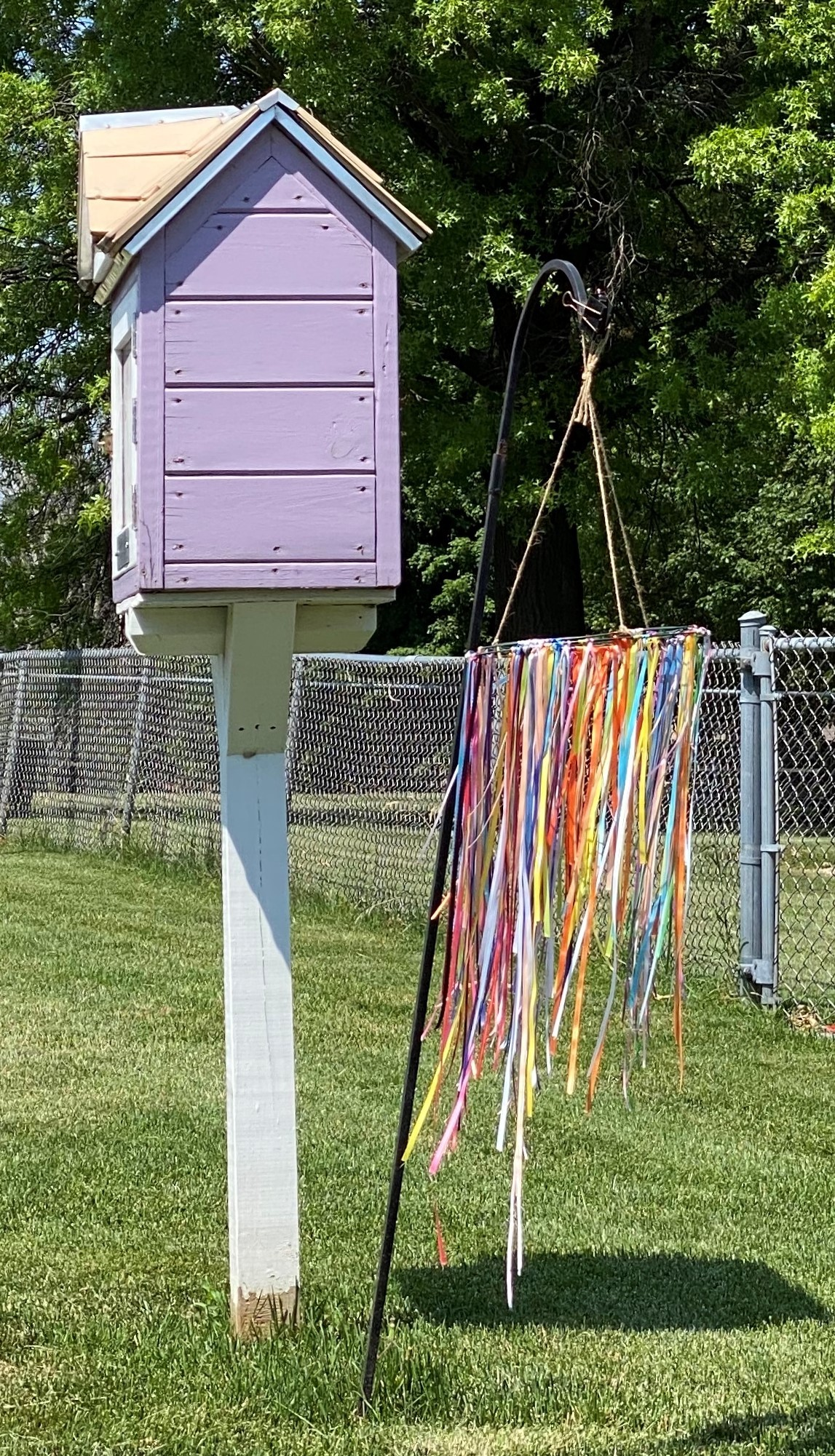Add a Ribbon, Say a Prayer!
Every year of Crop Walk we go past a house that has one of these cool, ribbon displays blowing in the wind. Please add a ribbon for someone or something in your prayers. While there check out our little free library!

 table div table+table+table+table+table+table+table+table+table+table+table+table+table+table+table+table+table+table+table div table{width:100%;padding:0}table div table+table+table+table+table+table+table+table+table+table+table+table+table+table+table+table+table+table+table div table img{width:96.23%;padding:0;float:none}table div table+table+table+table+table+table+table+table+table+table+table+table+table+table+table+table+table+table+table div table td{width:100%;padding:0 1.88% 18px}/* styles */St. Mark's School Uniform project is gearing up to again sell uniforms at Ellis, Lewis Lemon, and McIntosh Elementary Schools. Our inventory of new uniforms is complete, but we need more used uniforms. If you have used khaki or navy uniform pants sizes 5 through 18 or used uniform shirts in navy, light blue, yellow, red, or hunter green in sizes 5 through 18, please bring them to the St. Mark office. Not all parents can afford new uniforms for their children and are really appreciative of these used uniforms. Thanks for helping out! Questions? Call Stella Schroeder at 815-262-0938.

 table div table+table+table+table+table+table+table+table+table+table+table+table+table+table+table+table+table+table+table+table+table div table{width:100%;padding:0}table div table+table+table+table+table+table+table+table+table+table+table+table+table+table+table+table+table+table+table+table+table div table img{width:96.23%;padding:0;float:none}table div table+table+table+table+table+table+table+table+table+table+table+table+table+table+table+table+table+table+table+table+table div table td{width:100%;padding:0 1.88% 18px}/* styles */Betty Lundvall has moved to...
Heritage Woods Belvidere #120
Belvidere, Illinois 61008

Blessings on her move!

 table div table+table+table+table+table+table+table+table+table+table+table+table+table+table+table+table+table+table+table+table+table+table+table div table{width:100%;padding:0}table div table+table+table+table+table+table+table+table+table+table+table+table+table+table+table+table+table+table+table+table+table+table+table div table img{width:96.23%;padding:0;float:none}table div table+table+table+table+table+table+table+table+table+table+table+table+table+table+table+table+table+table+table+table+table+table+table div table td{width:100%;padding:0 1.88% 18px}/* styles */Wednesday, August 5, 12-3:00 P.M. at St. Mark. We are participating in “lemonade days!” We will have Drive-thru and walk-up!

FREE lemonade with hopes of donations to the Foundation for Childhood Cancer Research.

There will be hearts to write the name to honor or in memory of someone who is battling cancer. Please join us!

 table div table+table+table+table+table+table+table+table+table+table+table+table+table+table+table+table+table+table+table+table+table+table+table+table+table div table{width:100%;padding:0}table div table+table+table+table+table+table+table+table+table+table+table+table+table+table+table+table+table+table+table+table+table+table+table+table+table div table img{width:96.23%;padding:0;float:none}table div table+table+table+table+table+table+table+table+table+table+table+table+table+table+table+table+table+table+table+table+table+table+table+table+table div table td{width:100%;padding:0 1.88% 18px}/* styles */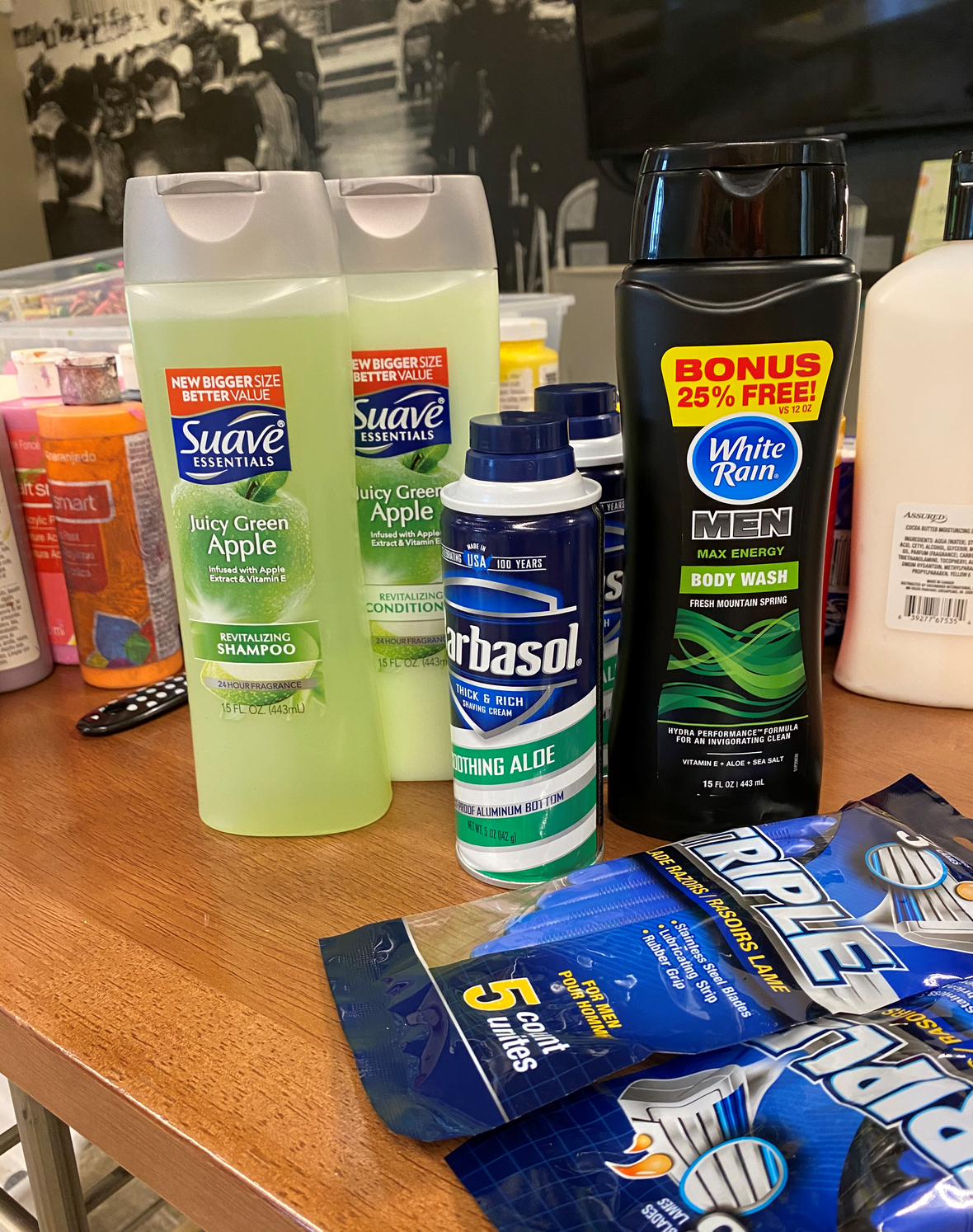The youth ministry team will be collecting at the end of August for...

Miss Carly’s Bath and Body type products, dry shampoo, and mini hand sanitizers, cloth face masks.

Unaccompanied Youth Project (Youth who do not have a consistent roof over their head) New cosmetic bags, men's toiletry bags, hair ties, stretch hairbands, wash, hand and bath towel and face masks.

Teacher CARE packages, monetary donations.

ALL these items will be collected the end of August. Watch for more details. Need more information now, e-mail melinda@stmarklc.com

 table div table+table+table+table+table+table+table+table+table+table+table+table+table+table+table+table+table+table+table+table+table+table+table+table+table+table+table div table{width:100%;padding:0}table div table+table+table+table+table+table+table+table+table+table+table+table+table+table+table+table+table+table+table+table+table+table+table+table+table+table+table div table img{width:96.23%;padding:0;float:none}table div table+table+table+table+table+table+table+table+table+table+table+table+table+table+table+table+table+table+table+table+table+table+table+table+table+table+table div table td{width:100%;padding:0 1.88% 18px}/* styles */A card or a call can make a person's week!
We, the church, you, are doing a good job of reaching out to others. Please continue to send cards, and make phone calls to our church community, neighbors, family, and friends. If there is someone you’ve been missing or wondering how they are, now is the time to reach out.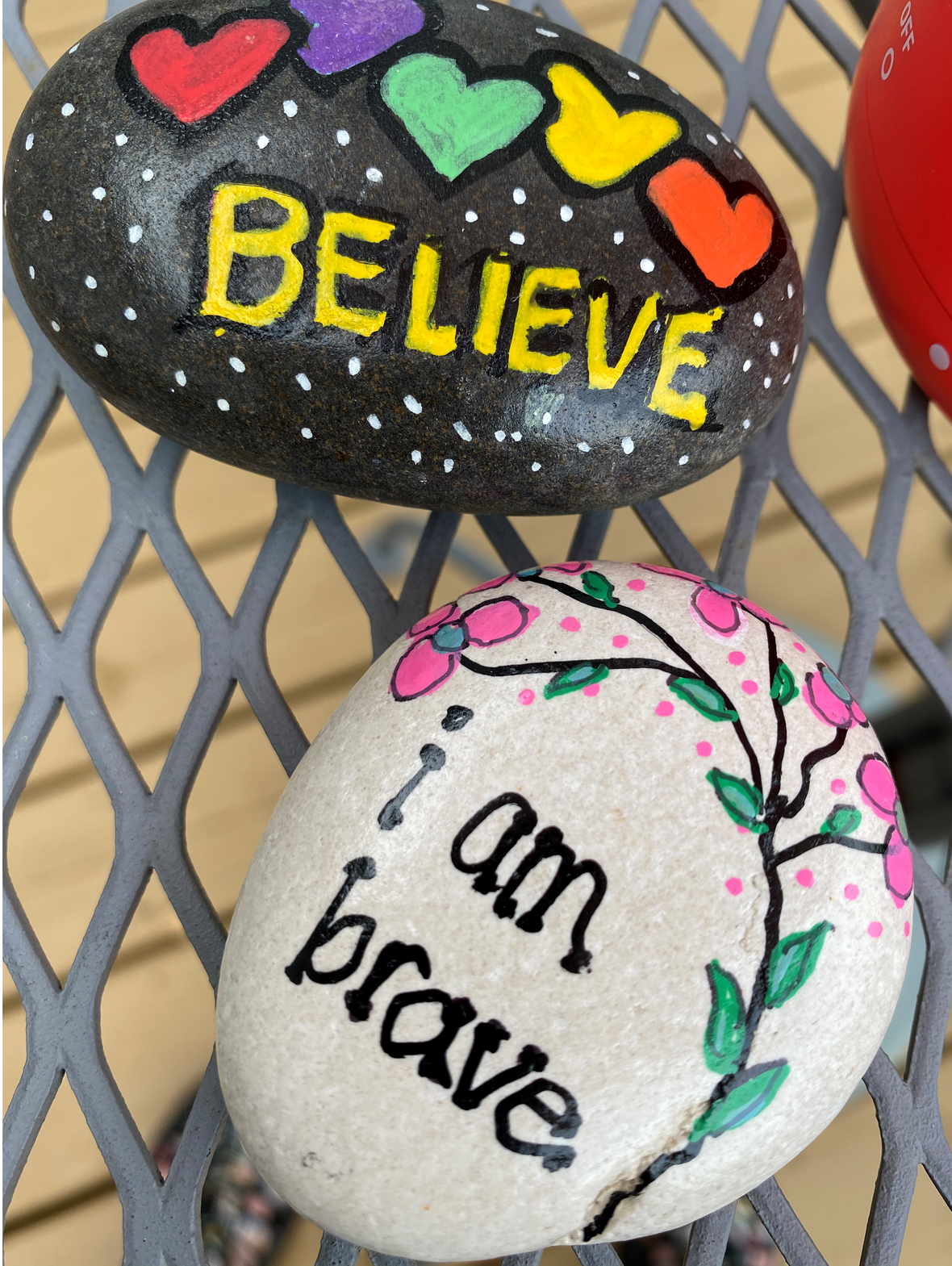table div table+table+table+table+table+table+table+table+table+table+table+table+table+table+table+table+table+table+table+table+table+table+table+table+table+table+table+table+table+table div table{width:100%;padding:0}table div table+table+table+table+table+table+table+table+table+table+table+table+table+table+table+table+table+table+table+table+table+table+table+table+table+table+table+table+table+table div table img{width:96.23%;padding:0;float:none}table div table+table+table+table+table+table+table+table+table+table+table+table+table+table+table+table+table+table+table+table+table+table+table+table+table+table+table+table+table+table div table td{width:100%;padding:0 1.88% 18px}/* styles */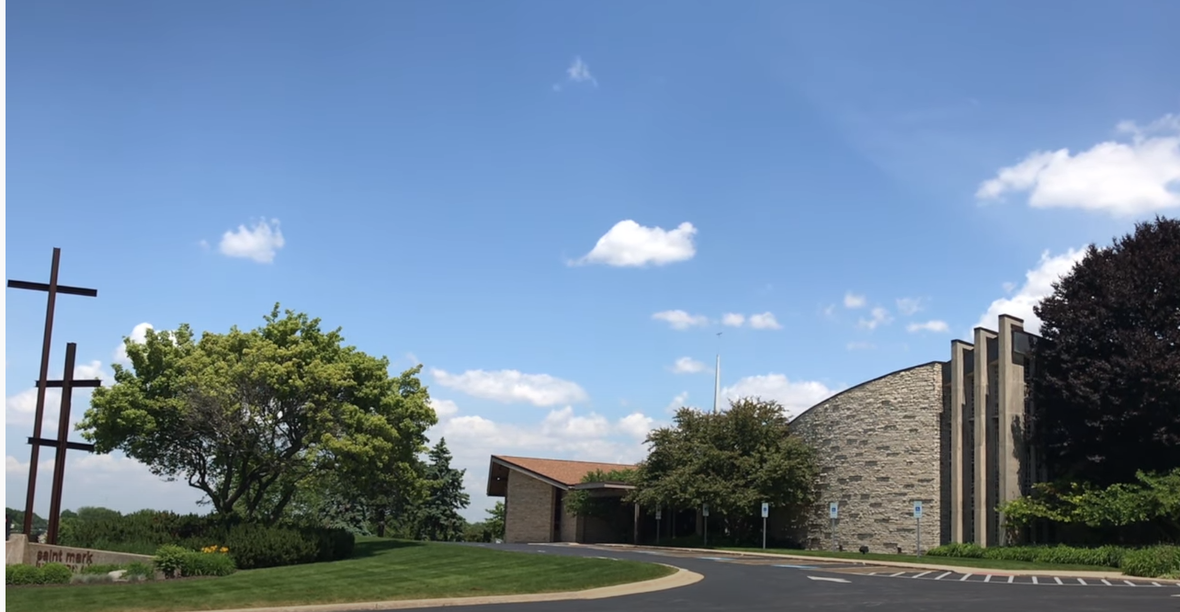Small Group Strategy & Guidelines
The council approved a motion to allow small groups to once again meet on church property. This is not a decision they have made lightly. Beginning July 1st, any groups that want to meet will be required to follow strict social distancing guidelines, and all participants will be asked to sign a waiver. This may sound extreme, but the recent uptick in cases around the country and even here in Illinois are a clear indication that we need to continue in our diligence to protect one another from this virus. Still, it is a welcome thing to be able to come to the building, and the council and we are certain we are able to gather safely, so long as we stick to the guidelines that have been given to us.Click here to read the guidelines.

 table div table+table+table+table+table+table+table+table+table+table+table+table+table+table+table+table+table+table+table+table+table+table+table+table+table+table+table+table+table+table+table+table div table{width:100%;padding:0}table div table+table+table+table+table+table+table+table+table+table+table+table+table+table+table+table+table+table+table+table+table+table+table+table+table+table+table+table+table+table+table+table div table img{width:96.23%;padding:0;float:none}table div table+table+table+table+table+table+table+table+table+table+table+table+table+table+table+table+table+table+table+table+table+table+table+table+table+table+table+table+table+table+table+table div table td{width:100%;padding:0 1.88% 18px}/* styles */Looking to volunteer yourself, as a family? There is a great opportunity locally at the Northern Illinois Food Bank. Check out solve hunger today.org There are times and dates Monday through Saturday.

 table div table+table+table+table+table+table+table+table+table+table+table+table+table+table+table+table+table+table+table+table+table+table+table+table+table+table+table+table+table+table+table+table+table+table div table{width:100%;padding:0}table div table+table+table+table+table+table+table+table+table+table+table+table+table+table+table+table+table+table+table+table+table+table+table+table+table+table+table+table+table+table+table+table+table+table div table img{width:96.23%;padding:0;float:none}table div table+table+table+table+table+table+table+table+table+table+table+table+table+table+table+table+table+table+table+table+table+table+table+table+table+table+table+table+table+table+table+table+table+table div table td{width:100%;padding:0 1.88% 18px}/* styles */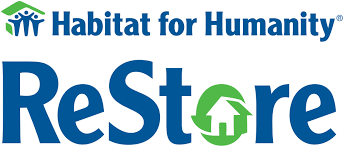Habitat ReStore Is Looking for Volunteers
Are you looking for a fun volunteer opportunity that will get you out of the house, while keeping you in a safe environment? The Rockford area Habitat for Humanity ReStore, located across from CherryVale mall, is looking for a few volunteers to help sort antique and vintage donated items.

Volunteers can work whatever hours fit their schedules, and the number of hours they volunteer each week is up to them. They will be trained on-site, will find the experience interesting and worthwhile as they support Habitat for Humanity here in town.

Sound interesting? Have questions?
Call or email John Hargrove, cell #815-289-0154, email jchargrove1116@gmail.com
St. Mark member and Habitat volunteer

 table div table+table+table+table+table+table+table+table+table+table+table+table+table+table+table+table+table+table+table+table+table+table+table+table+table+table+table+table+table+table+table+table+table+table+table+table div table{width:100%;padding:0}table div table+table+table+table+table+table+table+table+table+table+table+table+table+table+table+table+table+table+table+table+table+table+table+table+table+table+table+table+table+table+table+table+table+table+table+table div table img{width:96.23%;padding:0;float:none}table div table+table+table+table+table+table+table+table+table+table+table+table+table+table+table+table+table+table+table+table+table+table+table+table+table+table+table+table+table+table+table+table+table+table+table+table div table td{width:100%;padding:0 1.88% 18px}/* styles */St. Mark Mobile App!

This is different than our church directory app.
Our mobile app is designed specifically for St. Mark to improve our communication with our congregation and nurture our members. We encourage you to download the app. It's about getting involved and staying connected.

 table div table+table+table+table+table+table+table+table+table+table+table+table+table+table+table+table+table+table+table+table+table+table+table+table+table+table+table+table+table+table+table+table+table+table+table+table+table+table div table{width:100%;padding:0}table div table+table+table+table+table+table+table+table+table+table+table+table+table+table+table+table+table+table+table+table+table+table+table+table+table+table+table+table+table+table+table+table+table+table+table+table+table+table div table img{width:96.23%;padding:0;float:none}table div table+table+table+table+table+table+table+table+table+table+table+table+table+table+table+table+table+table+table+table+table+table+table+table+table+table+table+table+table+table+table+table+table+table+table+table+table+table div table td{width:100%;padding:0 1.88% 18px}/* styles */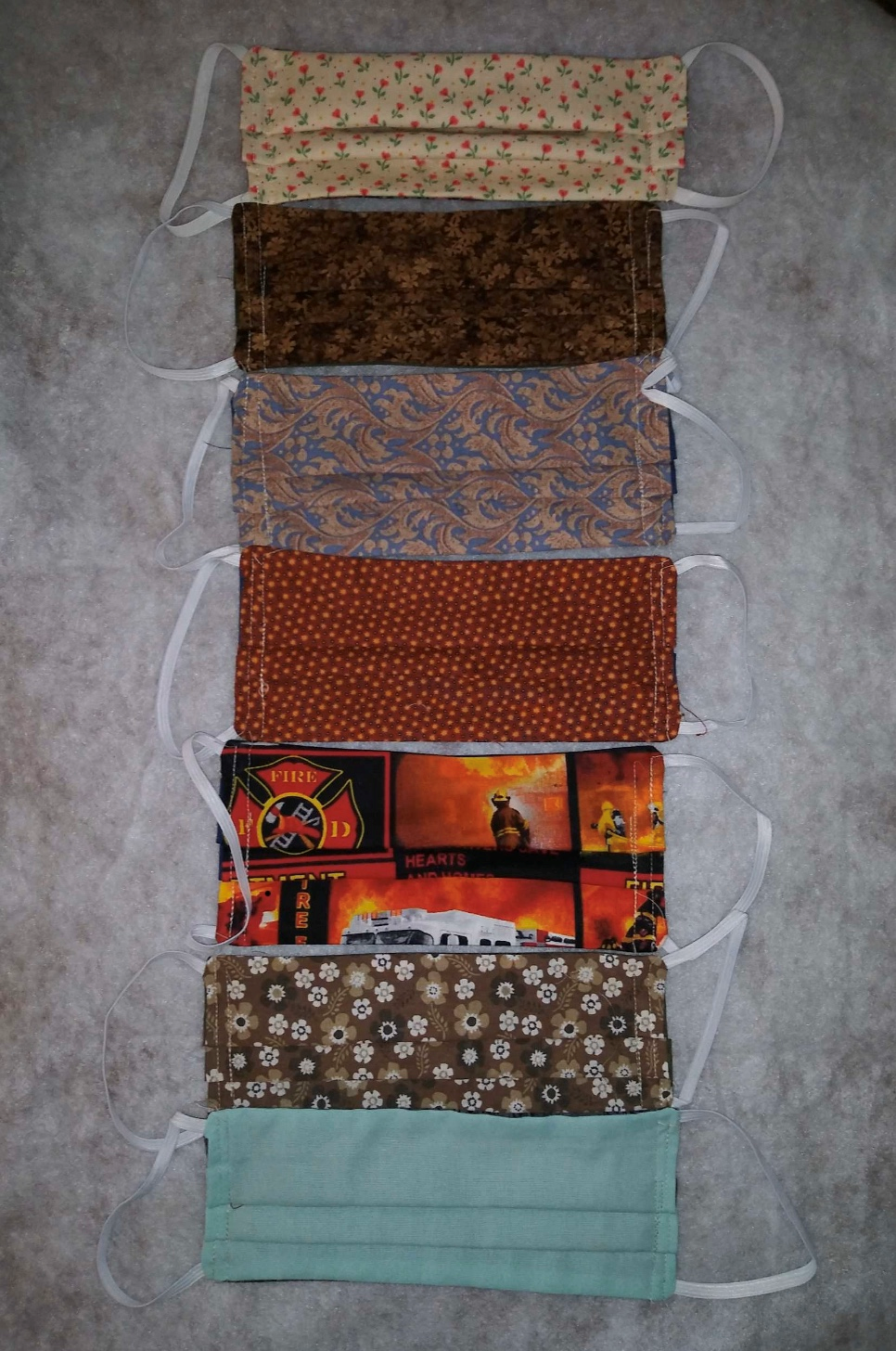Thank you to the Sew and Sews who have been incredibly busy. They have made 100’s of face masks. St. Mark is truly blessed by our Sew and Sews! Because of their generosity, their heart, and skills, the sewers are making masks for the congregation, as needed.

There is a bin of masks, outside the south doors. Monday, Wednesday, and Friday from 10:00 A.M. till Noon. If you need masks dropped to your residence, children size, or questions text/call Melinda Alekna 815-871-0390. If you would like to help with delivering to people, we could use your help as well.

 table div table+table+table+table+table+table+table+table+table+table+table+table+table+table+table+table+table+table+table+table+table+table+table+table+table+table+table+table+table+table+table+table+table+table+table+table+table+table+table+table div table{width:100%;padding:0}table div table+table+table+table+table+table+table+table+table+table+table+table+table+table+table+table+table+table+table+table+table+table+table+table+table+table+table+table+table+table+table+table+table+table+table+table+table+table+table+table div table img{width:96.23%;padding:0;float:none}table div table+table+table+table+table+table+table+table+table+table+table+table+table+table+table+table+table+table+table+table+table+table+table+table+table+table+table+table+table+table+table+table+table+table+table+table+table+table+table+table div table td{width:100%;padding:0 1.88% 18px}/* styles */St. Mark Prayer Chain
Would you like to be a part of the St. Mark prayer chain? Prayer requests come into the church office weekly. As we receive prayer requests we would send out an e-mail and ask that you pray daily for the persons mentioned in the requests. All requests are to remain confidential. Please e-mail melinda@stmarklc.com if you would like to participate in the prayer chain. “Be anxious for nothing, but in everything by prayer and supplication, with thanksgiving, let your requests be made known to God.” Colossians 4:2.

 table div table+table+table+table+table+table+table+table+table+table+table+table+table+table+table+table+table+table+table+table+table+table+table+table+table+table+table+table+table+table+table+table+table+table+table+table+table+table+table+table+table+table div table{width:100%;padding:0}table div table+table+table+table+table+table+table+table+table+table+table+table+table+table+table+table+table+table+table+table+table+table+table+table+table+table+table+table+table+table+table+table+table+table+table+table+table+table+table+table+table+table div table img{width:96.23%;padding:0;float:none}table div table+table+table+table+table+table+table+table+table+table+table+table+table+table+table+table+table+table+table+table+table+table+table+table+table+table+table+table+table+table+table+table+table+table+table+table+table+table+table+table+table+table div table td{width:100%;padding:0 1.88% 18px}/* styles */Instant Church Directory
This is a great time to stay connected with our St. Mark family. If you need help downloading the app or creating an account on your computer or ipad, please call Jill Davenport at 815-218-3403 or email jill@stmarklc.com and she will be happy to help you through the process.

It is simple and takes only a few minutes to upload your photo and download or create an online account on your computer or kindle. The Instant Church Directory App. You will always have updated members' information at your fingertips.

Instant Church Directory

Mobile App Login Instructions for Android & Kindle Devices

We also have a form for you to complete to make sure we have the most current information for the directory. There are paper forms at church or click on the link to Church Directory Form

 table div table+table+table+table+table+table+table+table+table+table+table+table+table+table+table+table+table+table+table+table+table+table+table+table+table+table+table+table+table+table+table+table+table+table+table+table+table+table+table+table+table+table+table+table div table{width:100%;padding:0}table div table+table+table+table+table+table+table+table+table+table+table+table+table+table+table+table+table+table+table+table+table+table+table+table+table+table+table+table+table+table+table+table+table+table+table+table+table+table+table+table+table+table+table+table div table img{width:96.23%;padding:0;float:none}table div table+table+table+table+table+table+table+table+table+table+table+table+table+table+table+table+table+table+table+table+table+table+table+table+table+table+table+table+table+table+table+table+table+table+table+table+table+table+table+table+table+table+table+table div table td{width:100%;padding:0 1.88% 18px}/* styles */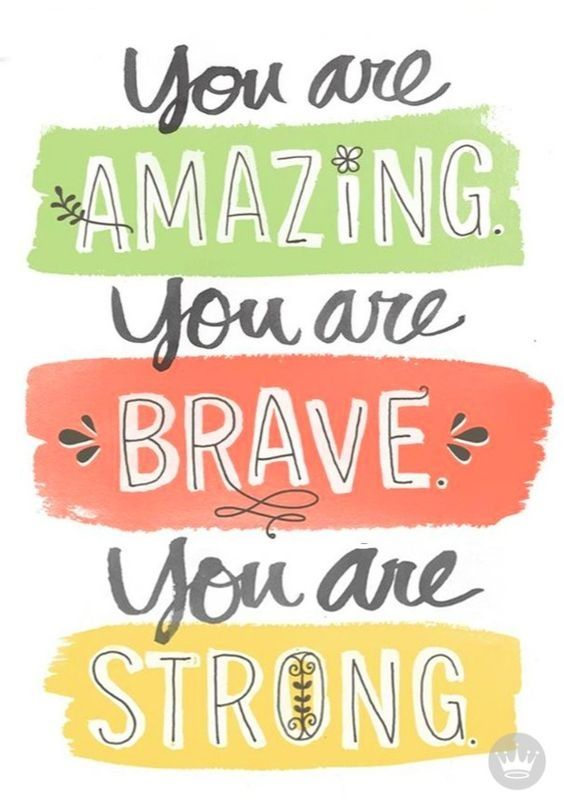table div table+table+table+table+table+table+table+table+table+table+table+table+table+table+table+table+table+table+table+table+table+table+table+table+table+table+table+table+table+table+table+table+table+table+table+table+table+table+table+table+table+table+table+table+table+table div table{width:100%;padding:0}table div table+table+table+table+table+table+table+table+table+table+table+table+table+table+table+table+table+table+table+table+table+table+table+table+table+table+table+table+table+table+table+table+table+table+table+table+table+table+table+table+table+table+table+table+table+table div table img{width:96.23%;padding:0;float:none}table div table+table+table+table+table+table+table+table+table+table+table+table+table+table+table+table+table+table+table+table+table+table+table+table+table+table+table+table+table+table+table+table+table+table+table+table+table+table+table+table+table+table+table+table+table+table div table td{width:100%;padding:0 1.88% 18px}/* styles */## Our St. Mark members:

Marilyn Vivian, Betty Adams, Sherri Lindquist, Tim Vivian, Bill Jennings, Tim Panjkovich, Bette Patterson, Jerry Cazaniga, David Young, Keith Haggestad.

## Family and friends of St. Mark members:

Kim Menke, Kevin Fleischer, Milane Bagel, Gayle Coffler, Darold, Beekmann, Janet Webster, Rae Ann McIntire, Linda Johnson, Nancy Kunnert, Robert and Cindy Wilson, Pastor Jane McChesney, Emily Tropp, Louis Fivaz, Mike Leonard, Katelynn Audette, Eva Love Sherbondy, Clay Heinrich, Norma Comstock, Carolyn King, Ashton Henley, Nathan Gyllin.

## Those serving in the military and their families:

Hunter Haggestad, Tristan Davenport, Scot Hornick, Bailey Panjkovich, Xander Gehrke, Jim Tammen, Brandon Ballenger, Taylor Eversole, Dean Barron.

Strengthen and encourage those in public health services and in the medical profession: care-givers, nurses, attendants, doctors, all who commit themselves to care for the sick and their families.

 table div table+table+table+table+table+table+table+table+table+table+table+table+table+table+table+table+table+table+table+table+table+table+table+table+table+table+table+table+table+table+table+table+table+table+table+table+table+table+table+table+table+table+table+table+table+table+table+table+table div table{width:100%;padding:0}table div table+table+table+table+table+table+table+table+table+table+table+table+table+table+table+table+table+table+table+table+table+table+table+table+table+table+table+table+table+table+table+table+table+table+table+table+table+table+table+table+table+table+table+table+table+table+table+table+table div table img{width:96.23%;padding:0;float:none}table div table+table+table+table+table+table+table+table+table+table+table+table+table+table+table+table+table+table+table+table+table+table+table+table+table+table+table+table+table+table+table+table+table+table+table+table+table+table+table+table+table+table+table+table+table+table+table+table+table div table td{width:100%;padding:0 1.88% 18px}/* styles *//* styles */ Office Hours Monday through Friday 9:00 A.M. - 1:00 P.M. Telephone: 815-398-3557 Publications St. Mark Memos, the weekly e-newsletter, is distributed each Thursday. The deadline for submission is Wednesday by 4:00 P.M. Please click to send submissions. Sermons Online Miss a Sunday at St. Mark? Please visit our website and watch the sermons online. Please click to watch.